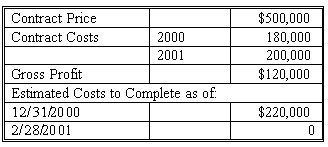### CFA Practice Question

There are 534 practice questions for this study session.

### CFA Practice Question

The following information applies to Smith Construction's Job 713 which was completed in 2001.Assuming Smith Construction uses the percentage-of-completion method of revenue recognition in accounting for Job 713, the gross profit recognized in 2000 would be ______.

A. \$100,000
B. \$45,000
C. \$36,000

The correct computation is as follows:
1) Percentage of completion as of at 12/31/00: 180,000/400,000 [180,000+220,000 estimate)=45% complete
2) Gross profit recognized in 2000: 45% x 100,000 est. GP [500,000-180,000-220,000] = 45,000

User Comment
shasha adjust total cost yearly based on adjusted estimated cost to complete.
quincy ok, I guess the estimated cost to complete as of 12/31/00 is the cost left for 2001, and since the estimated cost to complete as of 2/28/01 is 0 then cost completed in 2001 is 220000.
quincy does anyone know why the total cost is 180000 + 220000?
haarlemmer The prediction of the total costs was not accurate enough. Dut to that fact, the costs in 2001 is increased to 220k. In that sense, the total costs est. would be 180+220=400k and total gross profit is to be 100k. I hope this explains the question somehow.
mtcfa I think the question is fine. 180 is 45% of total estimated cost of \$400k. Take 45% of \$500k = \$225k. Then \$225k - 180k = \$45k.
ehc0791 Why can we assume the actual cost for 2000 is 160,000 ? 180,000 is the contract cost, not the actual cost. then for 2001, the estimated cost will be 220,000
treakj Timing is important. As of end of 2000, the estimated total cost at that time was 220,000. But in the end of 2001, the actual cost was 200,000. The percentage-of-completion method says that the cost that should be used is the estimated total cost (at that time), and if the estimated total cost changes over time, it does not change the revenue earned the year before.
itconcepts I think the catch here is remember that provision for losses are made immediately - so not only is the percentage completed less in the first year due to the new cost estimate for 2001, but ALSO it reduces the expected Total Gross Profit to 100,000
surob good question
lexology indeed
yonghui I see...
3dmouse Beware of timing,
t=0 YR2001
t=-1 YR2000
EST. COST ( 12/31/00) for the rest of the project which is in YR 2001 is 220,000
PLUS
INCURRED COST for YR2000 is 180,000
total RECENT est. cost = 400,000
% of completion is 180/400 = .45
rfvo YIP, Very good question, can see this one coming up in the exam.
sha09 the question was not very clear.
when it is indicating the contract cost, it should state the specific date...
azramirza Please help...check question 4 pertaining to cruise builders inc....it is on the same line but there estimated cost is ignored for calculating profit....why do we consider that here?
cleopatraliao I think I got it.To azramirza, always remember that % of completion=costs incurred to-date/most recent estimate of total costs. In Q4, the % of compltion=300,000/900,000 and the 900,000 is the estimated total coast. Here, they ask about the profit for 2000, so we don't know the real cost in 2001.Therefore,% of completion=180,000(cost incurred to-date)/(180,000+220,000)estimated total cost=45%. Since we know nothing abt the costs in 2001. So (ESTIMATED) Gross profit=0.45*(500,000-180,000-220,000)=45,000.
ranjansahay I think the question is not clear. It doesn't mention that 180k was already accrued for 2000, and 220k was a revised estimate for 2001. I assumed that in 2000, only 160k worth of work was done.
Shanax17 Good question!
johntan1979 After reading the question carefully again, it makes a lot of sense! It is asking for GP TO BE RECOGNIZED IN 2000, so the \$200,000 actual cost for 2001 is just a distraction, as well as the \$120,000 actual GP.
davcer Costs to complete, tricky
D3Er This project exceeded its internal budget